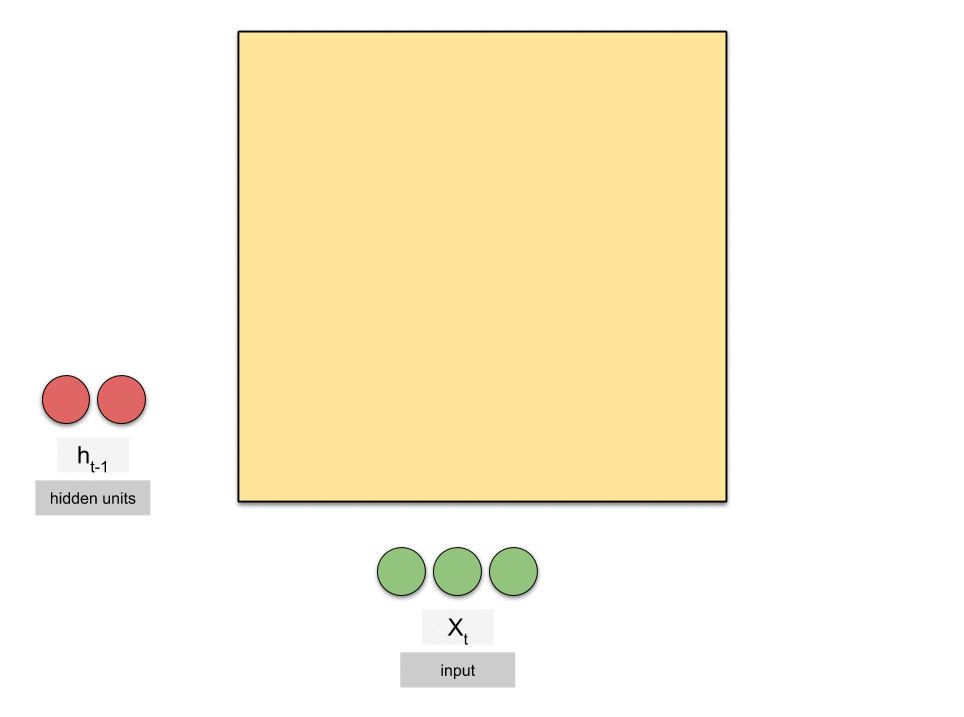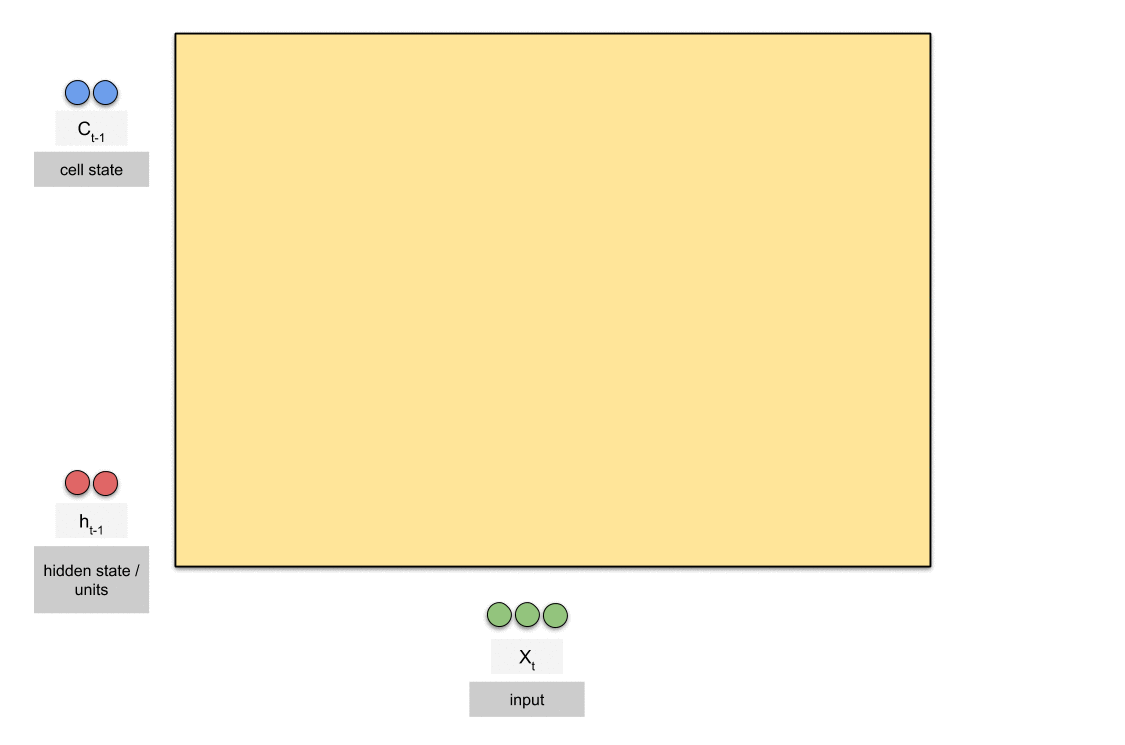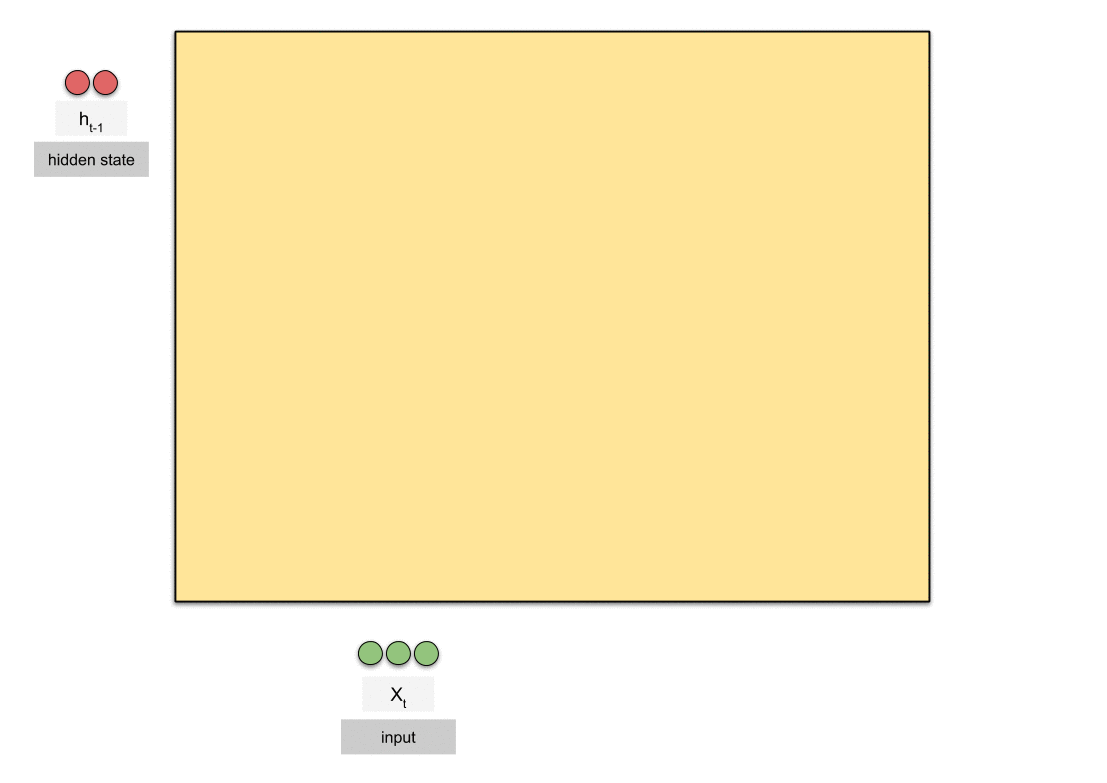Menu# Animated RNN, LSTM & GRU

Recurrent neural networks are a class of artificial neural networks which are often used with sequential data. The 3 most common types of recurrent neural networks are vanilla recurrent neural network (RNN), long short-term memory (LSTM) and gated recurrent units (GRU).

Here are the 3 GIFs (RNN, LSTM and GRU respectively) to help us understand what’s happening in each neural network. Note that they do not necessarily reflect the sequence of vectorised computations.You can read the full article at Towards Data Science here.

Share the post!

Related Posts